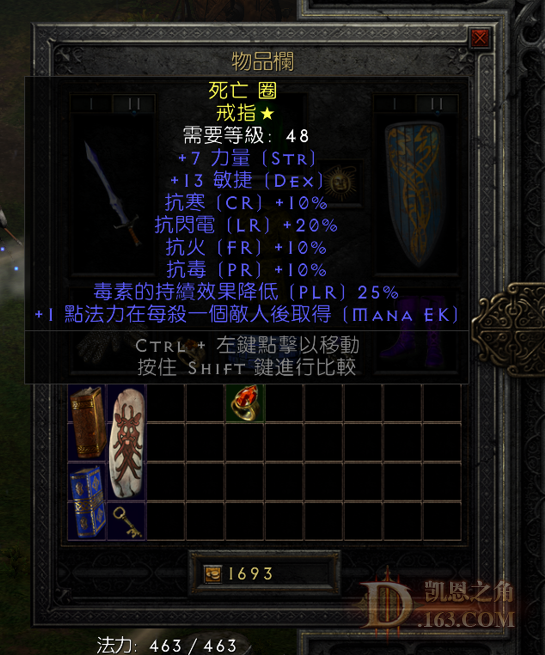//没登陆

 米山整天卡在后面，不帮我回血回蓝，典型的出工不出力。

33=0，32=0，31=0，30=0，29=0，28=0，27=3，26=0，25=0，24=2，格里风=0，次元=0，破隐=1，年纪=0，乔丹=2。
 51~60：大君，阿里巴巴，绿死人头。

33=0，32=0，31=0，30=0，29=0，28=0，27=3，26=0，25=0，24=2，格里风=0，次元=0，破隐=1，年纪=0，乔丹=2。
 黄黄黄 发表于 2022-5-7 11:44 米山整天卡在后面，不帮我回血回蓝，典型的出工不出力。 冲锋一起，他就不见了

 厉害啊楼主

 miniwalle 发表于 2022-5-6 17:52 这个是真实的。我也超市89级90%了，最大18#。 暗金出过一把丧钟一个物免盾。其他0 我88了16#以上的没见过

26#=2   27#=4   28#=0   29#=3
30#=3   31#=1   32#=0   33#=1
 60把地穴，从70级升到81级了，符文最大23，有没有达到凯恩平均水平呢？33=0，32=0，31=0，30=0，29=0，28=0，27=3，26=0，25=0，24=2，格里风=0，次元=0，破隐=1，年纪=0，乔丹=2。
 61~70：187大炮，蓝色次元（刚好可以拿来洗电棒）

33=0，32=0，31=0，30=0，29=0，28=0，27=3，26=0，25=0，24=2，格里风=0，次元=0，破隐=1，年纪=0，乔丹=2。
 71~80：空空如也。

33=0，32=0，31=0，30=0，29=0，28=0，27=3，26=0，25=0，24=2，格里风=0，次元=0，破隐=1，年纪=0，乔丹=2。
 多少mf？ zsbd

 多少MF啊 ，我80多了 ，一直再地穴，啥都不出啊

[armory]http://tw.battle.net/d3/zh/profile/Hanson-3389/hero/45661449[/armory]
 81~90：空。 感觉有点黑啊，现在268MF。

33=0，32=0，31=0，30=0，29=0，28=0，27=3，26=0，25=0，24=2，格里风=0，次元=0，破隐=1，年纪=0，乔丹=2。
 本帖最后由 黄黄黄 于 2022-5-8 00:27 编辑 91~100：18血9火炕小符，还有一个属性挺多的黄戒指。33=0，32=0，31=0，30=0，29=0，28=0，27=3，26=0，25=0，24=2，格里风=0，次元=0，破隐=1，年纪=0，乔丹=2。
 101~110：毛都没有！！！

33=0，32=0，31=0，30=0，29=0，28=0，27=3，26=0，25=0，24=2，格里风=0，次元=0，破隐=1，年纪=0，乔丹=2。
 111~120：还是空，有点过分了！33=0，32=0，31=0，30=0，29=0，28=0，27=3，26=0，25=0，24=2，格里风=0，次元=0，破隐=1，年纪=0，乔丹=2。
 从昨晚开始估计20把+，出了骑士套装头、风之力、28#。全身就精神剑（盾）和107防御的隐秘